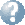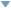Online Reference Dictionary, Encyclopedia & moreby: WordStarts with Ends withMentionsteaching:topics:calculus:limits

### Limits

$\newcommand{\defeq}{\stackrel{\scriptsize{\mathsf{def}}}{=}}$ To work with these infinite sums and come to some formal, mathematical, way of resolving the wolf question properly we should have a notation which does not involve ‘cdots’ or other vague and perhaps ambiguous ideas.

Rather than asking what the final sum is after adding up the whole of an infinite sequence of numbers (whatever that might actually mean) we look for a number that you can get as close to as you want by keeping on adding far enough along that series. The sum will never quite reach that value, but will get closer and closer the more tiny extra numbers we add. We call this value the “limit” of this sum.

We start with a new way of writing the expression for how far the wolf has run after 5 intervals, using a new operation $\sum\limits_{k=1}^{5}$ called “sum” which means adding together each of the values of the sequence made by setting (in this example) k to each integer from 1 to 5 in the expression (or function) after it … $W_5 = \sum_{k=1}^{5}\frac{200}{2^k}$ We are using this new variable, here k, like we use the index or counter in a computer program. Now we can talk about distance travelled after counting off n intervals in the wolf’s pursuit … $W_n = \sum_{k=1}^n\frac{200}{2^k}$ Lastly we want to be able to write the sum if we kept adding up and somehow (?!?) included all of the infinite number of smaller and smaller distances: $W = \sum_{k=1}^\infty\frac{200}{2^k}$ … then when we think carefully about this … we certainly want to havequad W=200 quadhere … we also want to think about different sums when the answer is not some nice, neat finite value … and we want to keep our use of $\large\infty$ carefully contained, where we can make some exact sense of it and we can be more careful about what we mean by phrases like “… will continue endlessly”.

So mathematicians invented another notation, and a new … very powerful! … idea to explore, we use notation like ‘$\ \lim\limits_{n\to\infty}\$' for the idea of the ‘limit’ we spoke about above, and start our exploration by defining: $\large\sum_{k=1}^{\infty}{\rm f}(k) \defeq \lim_{n\to\infty}\sum_{k=1}^n {\rm f}(k)$

#### Now take time to see what we can make of all this!

What do we mean when we write $\quad\lim\limits_{x\to n}{\rm f}(x)$ ?

Then … what does it mean to write $\quad\lim\limits_{x\to\infty}{\rm f}(x)$ ?

Now … try to graph the function: $\displaystyle\quad {\rm f}(x) = \frac{x^2-a^2}{x-a},\quad$ say when \ a = 2

What is the value of this text(f)(x) whenquad x=a ?

What do you think $\displaystyle\quad\lim\limits_{x\to a}\frac{x^2-a^2}{x-a}\quad$ should be?

What about $\quad\lim\limits_{x\to\infty}{\rm f}(x)\quad$ and $\quad\lim\limits_{x\to -\infty}{\rm f}(x)\$?

teaching/topics/calculus/limits.txt · Last modified: 2020/09/13 18:53 by simon

### Page Tools